# 【Python】NumPy数组和矢量计算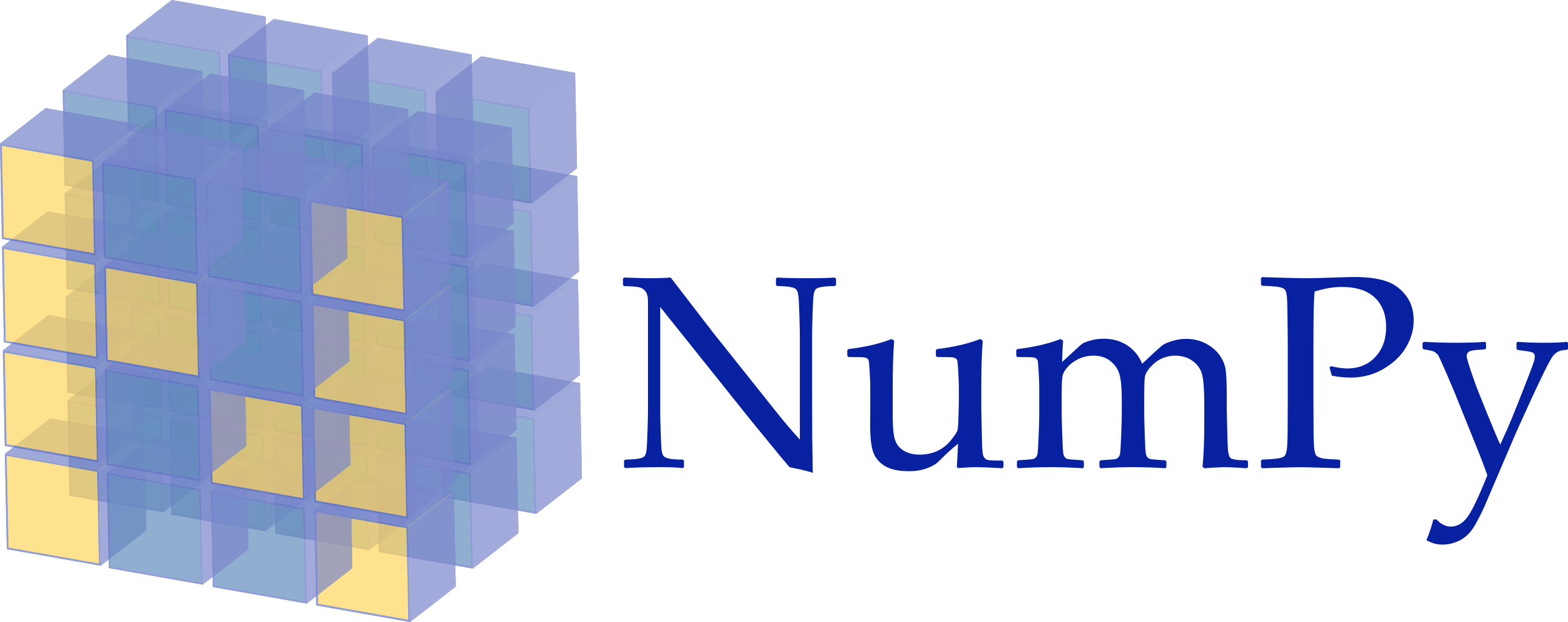1.NumPy安装：

2.检测安装是否成功：

1.array创建：

range的使用：

arange创建数组：

2.随机数创建：

dtype的使用：

3.创建正态分布数组：

1.zeros：

2.ones：

3.empty：

4.linspace：

5.logspace：

1.改变数组得到维度：

2.数组的拼接：

concatenate连接数组：

3.数组的分割：

4.数组的转置：

NumPy函数：

# 一、NumPy的ndarray：一种多维数组对象：NuPy（Numerical Python）是科学计算基础库，提供大量科学计算相关功能，比如数据统计，随机数生成等。

NumPy最重要的一个特点就是N维数组对象（ndarray），该对象是一个快速而灵活的大数据集容器。ndarray是一个通用的同构数据的多维容器，也就是说，ndarray中的所有元素必须是相同类型的数据。

## 1.NumPy安装：

``````pip install numpy
``````

## 2.检测安装是否成功：

``````import numpy as np

a = np.arange(10)

print(a)

# type()：返回当前参数的数据类型：
print(type(a))
``````# 二、数组创建：

## 1.array创建：

numpy模块的array函数可以生成多维数组。例如，如果要生成一个二维数组，需要向array函数传递一个列表类型的参数。每一个列表元素是一维的ndarray类型数组，作为二维数组的行。

``````import numpy as np

a = np.array([1, 2, 3, 4], dtype=float, ndmin=3)

print(a)
print(type(a))
``````
``````# NumPy中的ndarray对象：

# 导入NumPy模块
import numpy as np

# 定义一个NumPy的ndarray对象
data = np.array([[1, 2, 3, 4], [5, 6, 7, 8]], np.int32)

# 输出ndarray数组中元素数据类型
print(data.dtype)

# 输出ndarray对象结构
print(data.shape)
``````

• dtype：设置创建numpy数组的数据类型
• ndmin：设置创建numpy数组的维度

### range的使用：

range范围遵守左闭右开原则，包含start元素，不包含stop元素！

``````range(start, stop, step)
``````

### arange创建数组：

``````arange(start, stop, step, dtype)
``````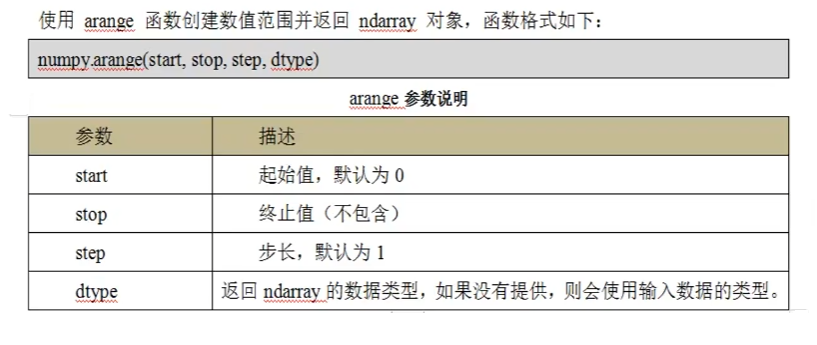## 2.随机数创建：

numpy.random.random(size = None)：该方法返回 [ 0.0，1.0）范围的随机数。size指定数组形状（元素个数）。

``````import numpy as np

a = np.random.random(size=10)

print(a)
print(type(a))
``````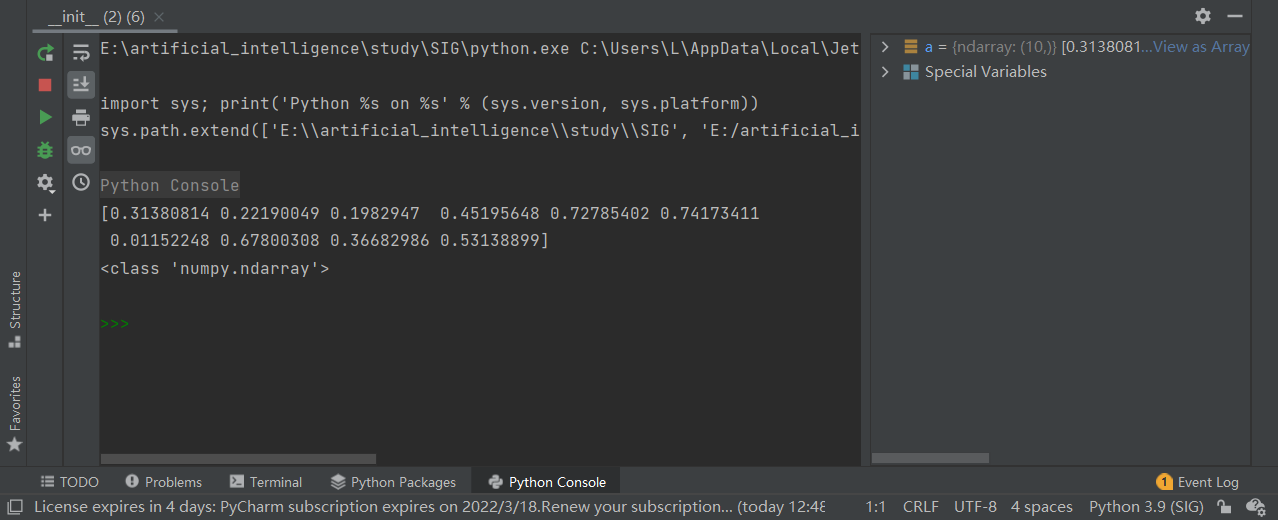``````import numpy as np

# 创建一个二维数组：
a = np.random.random(size=(2, 2))

print(a)
print(type(a))
``````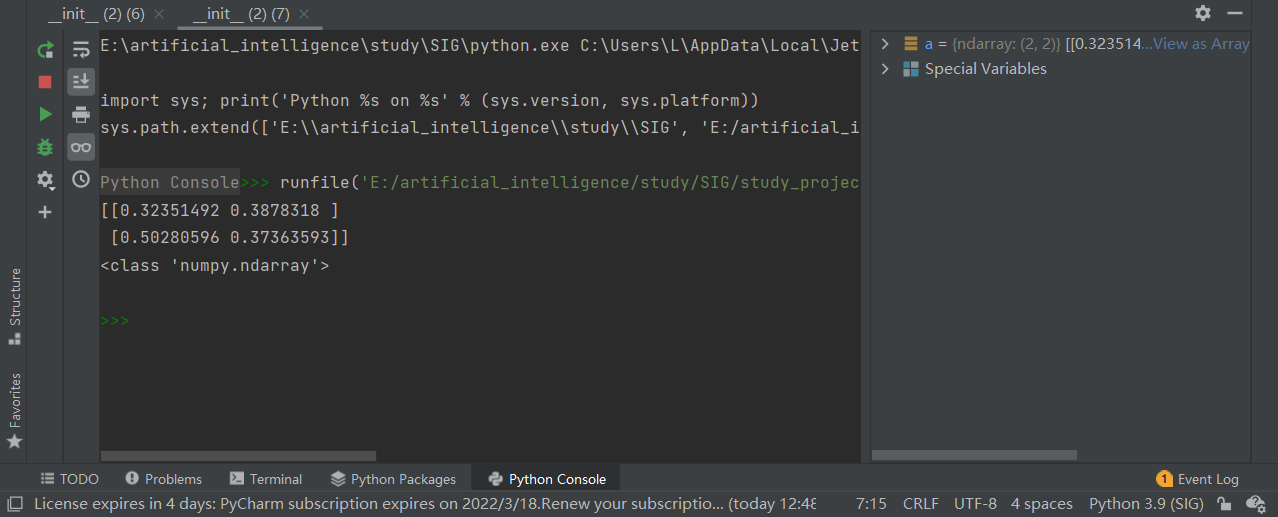### 随机整数：

``````np.random.randint(low, hight=None, size=None, dtype="1")
``````

### dtype的使用：

``````import numpy as np

# 使用dtype指定数据类型，np包含许多的数组类型
a = np.random.randint(0, 10, size=(2, 3), dtype=np.int64)

print(a)

# dtype属性：当前数组中的元素值类型
print(a.dtype)
``````## 3.创建正态分布数组：

randn函数返回一个或一组样本，具有标准正态分布（期望为0，方差为1）。dn表格每个维度。返回值为指定维度的array

``````np.random.randn(d0, d1, d2, ... ,dn)
``````
``````import numpy as np

# 创建标准的正态分布：
a = np.random.randn(2, 3, 4)

print(a)
``````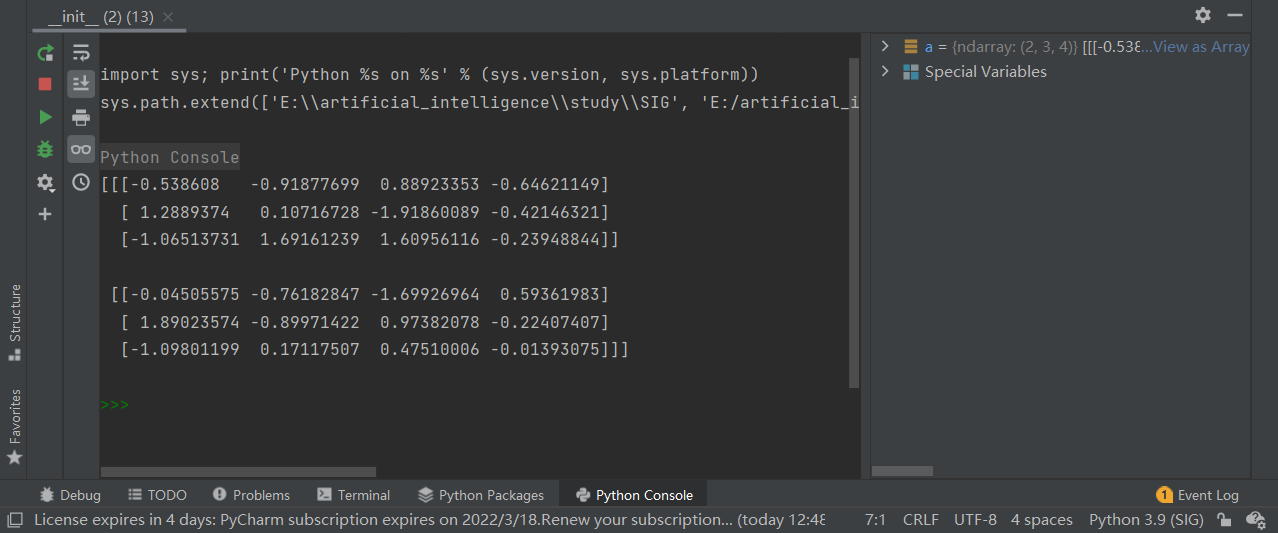### 创建指定期望和方差的正太分布：

``````import numpy as np

# normal：创建指定期望和方差的正态分布；默认期望：loc=0.0；方差scale=1.0
a = np.random.normal(0, 1, size=4)

print(a)
``````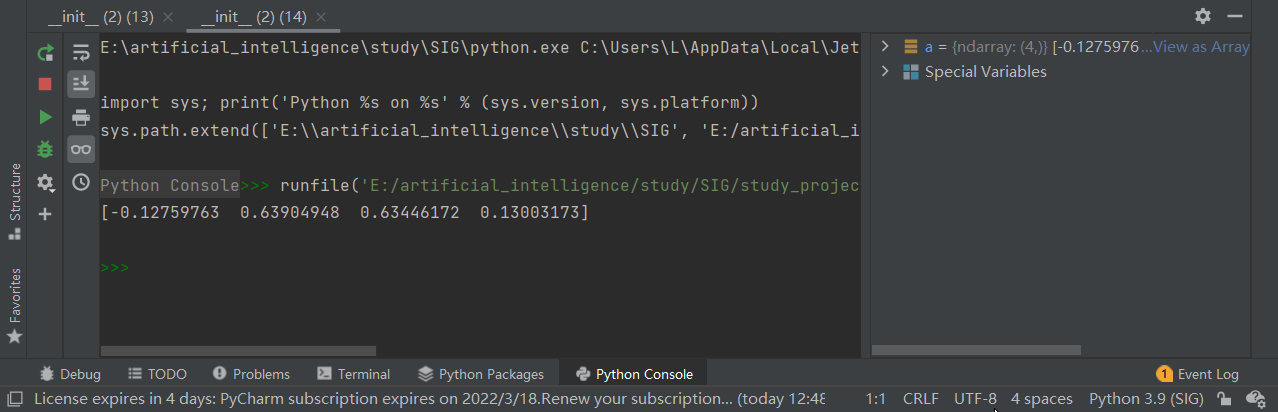# 三、ndarray对象：

NumPy最重要的一个特点是其N维数组对象 ndarray，它是一系列同类型数据的集合，以0下标为开始进行集合中元素的索引。

ndarray对象是用于存放同类型元素的多维数组。

ndarray中的每个元素在内存中都有相同存储大小的区域

ndarray内部由以下内容组成：

• 一个指向数据（内存或内存映射文件中的一块数据）的指针
• 数据类型或dtype，描述在数组中的固定大小值的格子。
• 一个表示数组形状（shape）的元组，表示各维度大小的元组

NumPy的数组中比较重要ndarray对象属性有：## 创建ndarray数组的其他方式：

ndarray数组除了可以使用底层的ndarray构造函数来创建以外，也可以通过以下几种方式创建。

### 1.zeros：

zeros创建指定大小的数组，数组元素以0来填充：

``````numpy.zeros(shape, dtype, order="C")
``````
``````import numpy as np

# 创建以0填充的数组：
a = np.zeros(shape=(2, 3), dtype=float, order="C")

print(a)
``````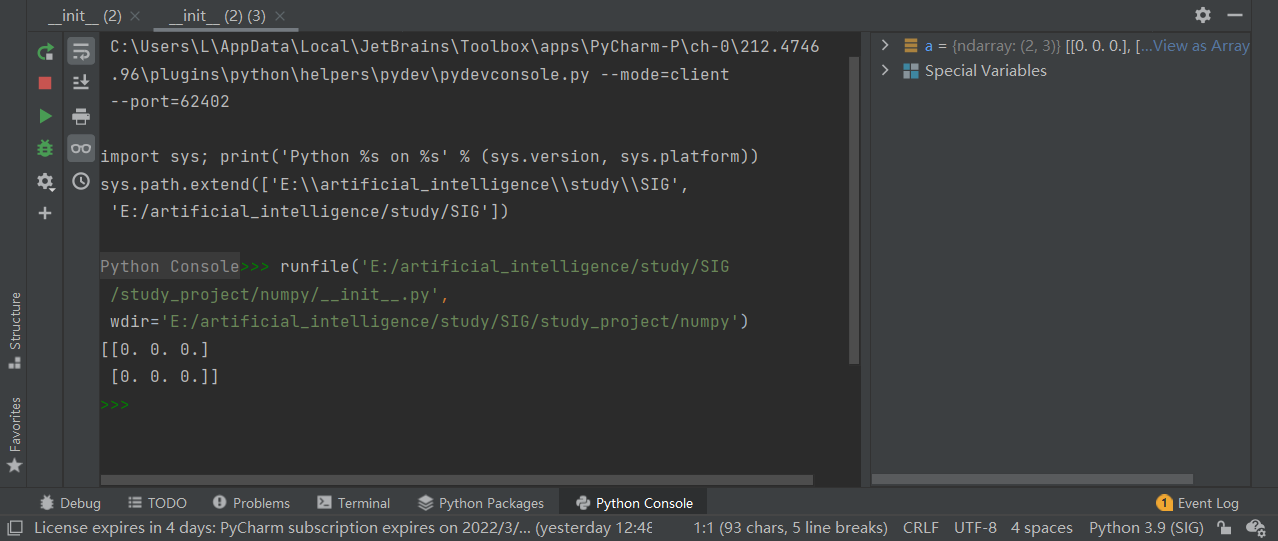### 2.ones：

numpy.ones：创建指定形状的数组，数组元素以1来填充：

``````numpy.ones(shape, dtype, order="C")
``````
``````import numpy as np

# 创建以1填充的数组：
a = np.ones(shape=(2, 3), dtype=float, order="C")

print(a)
``````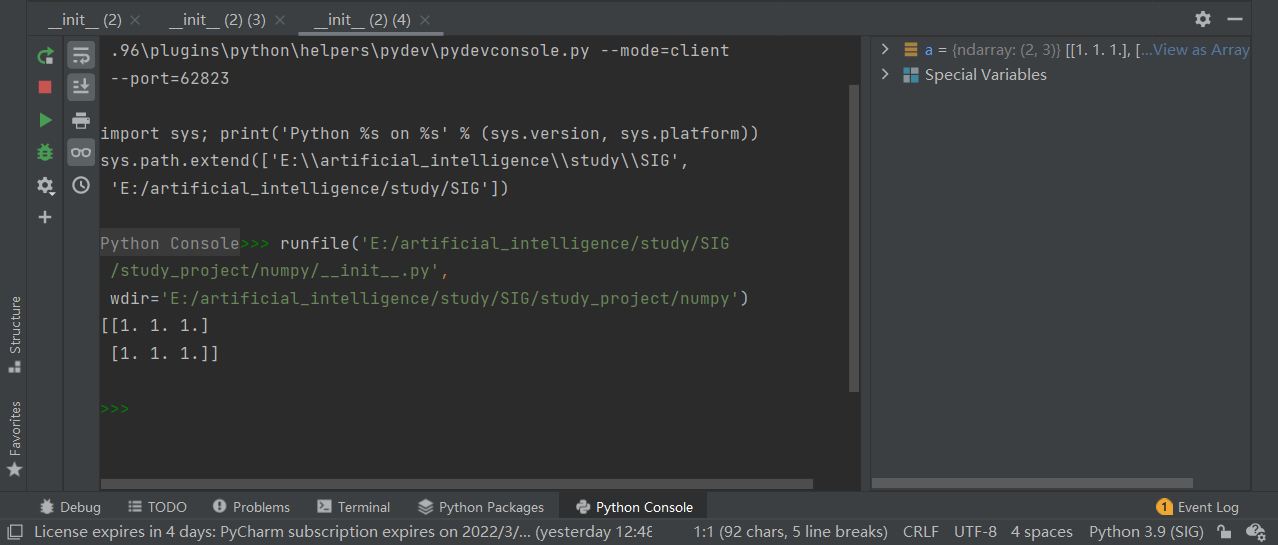### 3.empty：

numpy.empty方法用来创建一个指定形状（shape）、数据类型（dtype）且初始化的数组，里面的元素的值是之前内存的值：

``````numpy.empty(shape, dtype, order="C")
``````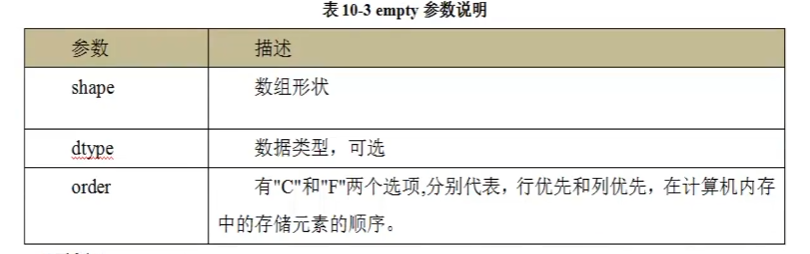### 4.linspace：

linspace函数用户创建一个一维数组，数组是一个等差数列构成的：

``````numpy.linspace(start, stop, num=50, endpoint=True, retstep=False, dtype=None)
``````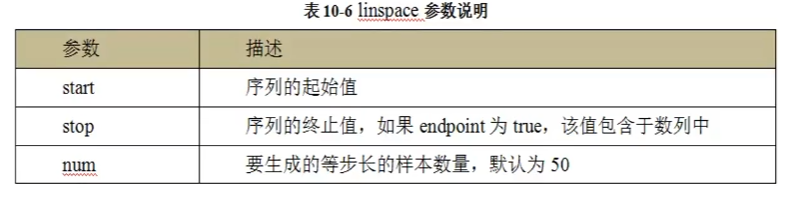• endpoint：是否包含结束数值
• retstep：是否显示生成的数组中间显示间距

### 5.logspace：

numpy.logspace函数用于创建一个等比数列：

``````numpy.logspace(start, stop, endpoint, base, dtype=None)
``````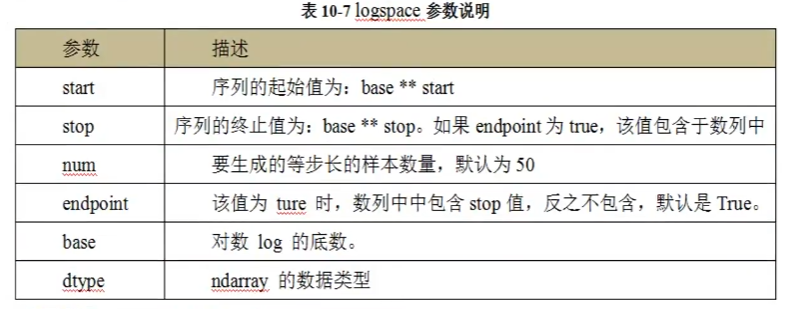# 四、切片和索引：

ndarray对象的内容可以通过索引或者切片来访问和修改，与Python中list的切片操作一样。

ndarray数组可以基于0-n的下标进行索引，并设置start，stop以及step参数进行，从原数组中割出一个新数组。

``````[start:stop:step]
``````

### 修改一维数组的形状：

``````array.reshap((&#x6570;&#x7EC4;&#x683C;&#x5F0F;))
``````
``````import numpy as np

a = np.arange(1, 11)

# 修改数组形状：
a = a.reshape((5, 2))

print(a)
``````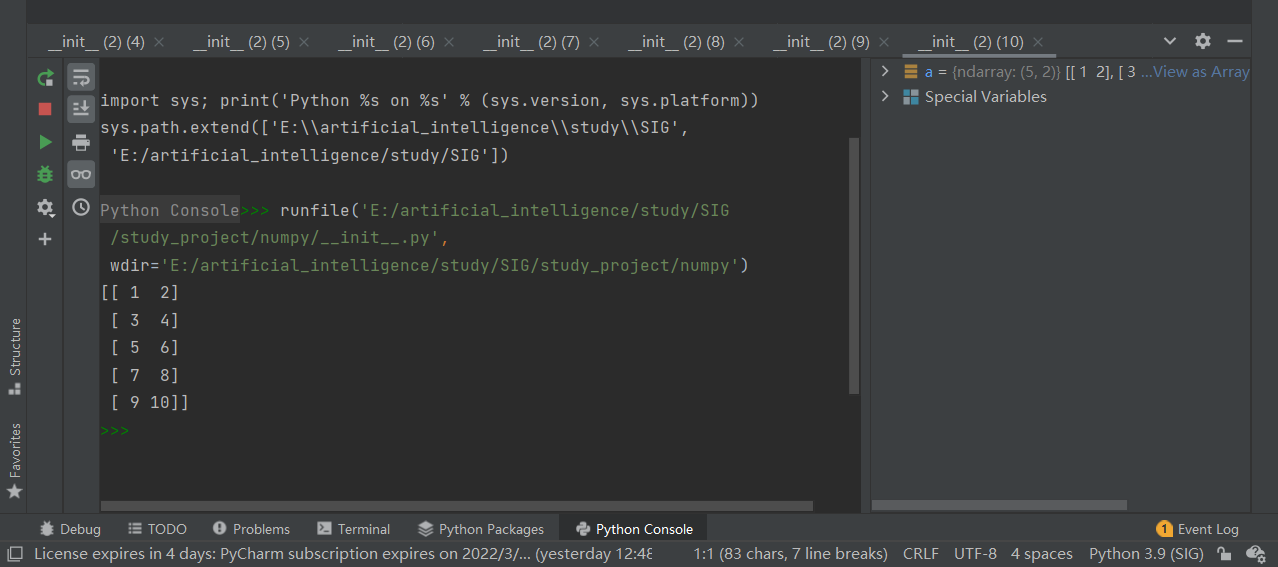## 数组的复制：

[En]

Even if the array taken from all the slices is assigned to a new variable, it still has a view of all the original arrays. (slicing is an address assignment, not value transfer. If you modify the element value in the sliced array, it will affect the original array)

``````numpy.copy(&#x539F;&#x6570;&#x7EC4;)
``````

# 五、数组的相关操作：

## 2.数组的拼接：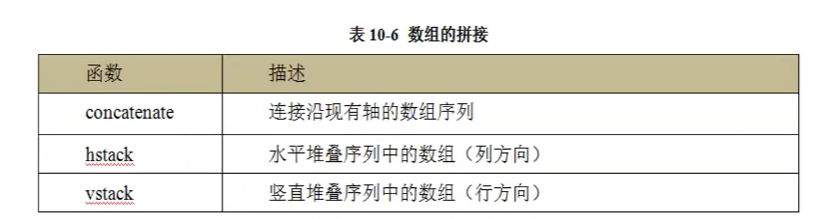### 水平方向拼接：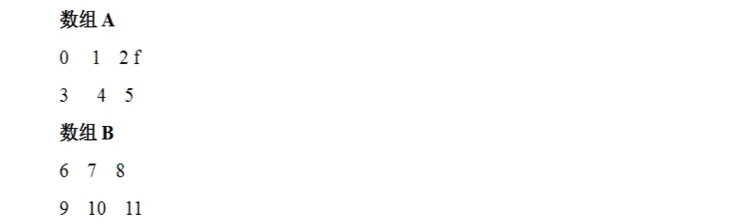``````numpy.hstack([A, B])
``````### 垂直方向拼接：

``````numpy.vstack((A, B))
``````

### concatenate连接数组：

``````numpy.concatenate((a1, a2, a3, ... ), axis)
``````

• a1，a2，......：相同类型的数组
• axis：沿着它连接数组的轴，默认为0

## 3.数组的分割：

numpy.spit函数沿着特定的轴将数组分割为子数组：

``````numpy.split(ary, indices_or_sections, axis)
``````

• ary：被分割的数组
• indices_or_sections：如果是一个整数，就用数平分切分，如果是一个数组，为沿轴切分的位置。
• axis：沿着哪个维度进行切向，默认为0，横向切分。为1时纵向切分。

## 4.数组的转置：

``````numpy.transpose()
``````

# NumPy函数：

## 统计函数：

[En]

Statistical function, which is used to find the minimum element, maximum element, percentile standard deviation and variance from the array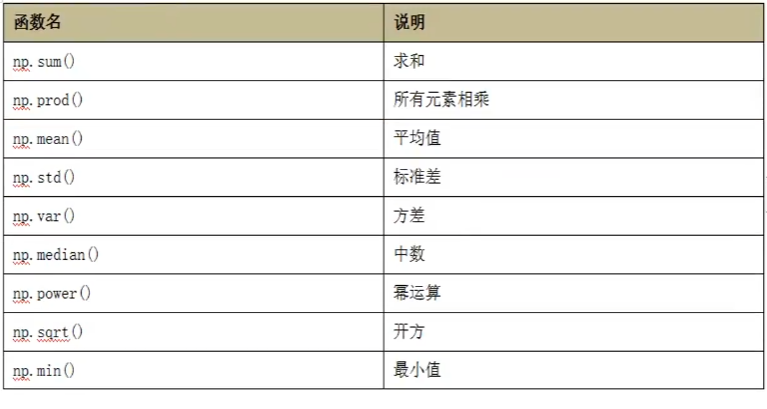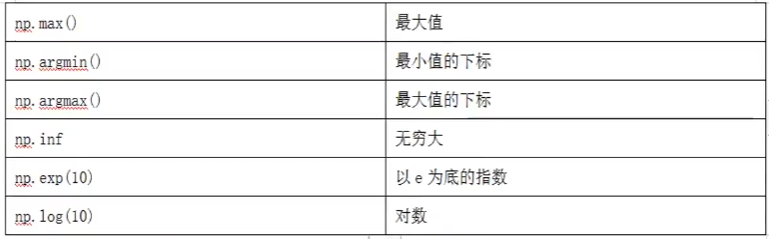Original: https://blog.csdn.net/weixin_52058417/article/details/122684904
Author: 爱吃糖的范同学
Title: 【Python】NumPy数组和矢量计算

## Title: Tensorflow 2.9.1安装笔记

CPU：i7-4790k

Cuda 版本：11.3

Cunn版本: 11.6

Python版本:3.7.7

1.准备工作

• 安装python3.7.7(之前安装好的)

deviceQuery.exe 和 bandwithTest.exe测试通过。

• 下载Tensorflow

• 安装组件

A.Numpy: pip install numpy -i https://pypi.tuna.tsinghua.edu.cn/simple

B.mkl: pip install mkl -i https://pypi.tuna.tsinghua.edu.cn/simple

C.protobuf pip install protobuf -i https://pypi.tuna.tsinghua.edu.cn/simple

2.安装Tensorflow

pip install tensorflow-2.9.1-cp37-cp37m-win_amd64.whl -i https://pypi.tuna.tsinghua.edu.cn/simple

3.测试

``````import tensorflow as tf
print("Tensorflow version:")
print(tf.__version__)

print("CPU/GPU devices for Tensorflow:")
gpus = tf.config.experimental.list_physical_devices(device_type='GPU')
cpus = tf.config.experimental.list_physical_devices(device_type='CPU')
print(gpus)
print(cpus)
``````

Tensorflow version:
2.9.1
CPU/GPU devices for Tensorflow:
[PhysicalDevice(name='/physical_device:GPU:0', device_type='GPU')]
[PhysicalDevice(name='/physical_device:CPU:0', device_type='CPU')]

IDE可以使用Visual Studio Code（小规模测试）

Original: https://blog.csdn.net/st01lsp/article/details/125294794
Author: st01lsp
Title: Tensorflow 2.9.1安装笔记

## 1.有关于在Windows下配置tensorflow和pytorch深度学习环境教程

tensorflow安装、pytorch安装 Nvidia显卡驱动安装 tensorflow 安装 pytorch安装 Nvidia显卡驱动安装 只要是带有Nvidia显卡的电脑基本上都是可以用来进行...

## opencv基本操作二（读取视频流与保存视频、读取摄像头并保存视频）

opencv基本操作二 要点 代码 * 例程一 例程二 要点 opencv常用 读视频函数 cv2.VideoCapture 、 cv2.VideoCapture.get 等，可以参考这里 openc...

## Matlab–k-means聚类算法实现

** Matlab–k-means聚类算法实现 ** 第一次在CSDN写自己的博客，希望开门大吉 哈哈哈 也希望分享的内容大家能够喜欢。 本次创作内容为-----k-means聚类算法实现。 自己用m...

## Bert模型学习之句向量的简单应用

Bert模型学习之预训练模型的简单应用 上文讲到，让自己的机器可以同时安装两个版本的python3.x，下面我们就可以正式运行Bert模型。 一、框架安装 1.首先切换到版本3.6的文件夹下 2.安装...

## LIO-SAM从0到1运行自己的数据集

LIO-SAM从0到1运行自己的数据集 前言 ​ 笔者在学习LIO_SAM时踩了不少坑，在此记录从开始到最后整个踩坑过程。文中参考了很多大佬的文章，我只是个搬运工。可以直接跳到第二部分从0到1实现 有...

## 知识图谱-Neo4j入门介绍和实践

Neo4j基本介绍 图数据库在社交网络、实时推荐、征信系统、人工智能等领域有广泛应用，知识图谱以图数据库作为存储引擎，对海量信息进行智能化处理，形成大规模的知识库并进而支撑业务应用。. 软件安装后的引...